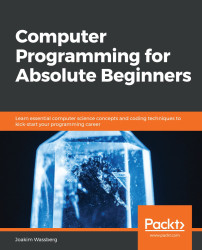•#### Computer Programming for Absolute Beginners#### Overview of this book

PrefaceSection 1: Introduction to Computer Programs and Computer ProgrammingFree Chapter
Chapter 1: Introduction to Computer ProgramsChapter 2: Introduction to Programming LanguagesChapter 3: Types of ApplicationsChapter 4: Software Projects and How We Organize Our CodeSection 2: Constructs of a Programming LanguageChapter 5: Sequence – The Basic Building Block of a Computer ProgramChapter 6: Working with Data – VariablesChapter 7: Program Control StructuresChapter 8: Understanding FunctionsChapter 9: When Things Go Wrong – Bugs and ExceptionsChapter 11: Programming Tools and MethodologiesSection 3: Best Practices for Writing High-Quality CodeChapter 12: Code QualityOther Books You May EnjoyAppendix A: How to Translate the Pseudocode into Real CodeAppendix B: Dictionary# Handling exceptions

To understand how we can handle exceptions, we must first understand what the origins of a problem might be. It is only when we have understood this that we can insert measures to handle them correctly.

Let's return to our function that divides two values. Let's say this function takes two arguments, as it did in our previous example:

```function c(x, y)
result = x / y
end_function```

We should assume that this function does something more than just print this single line. We can mark it out with some comments, as follows:

```function c(x, y)
// The function does some things ere
result = x / y
// And even more things here
// It might even return a value
end_function```

We know that as this function divides two values, we might get an exception if `y` is given a value of `0`.

The first thing we should ask ourselves is if this is the...